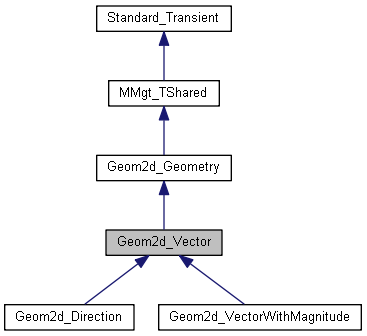# Geom2d_Vector Class Referenceabstract

The abstract class Vector describes the common behavior of vectors in 2D space. The Geom2d package provides two concrete classes of vectors: Geom2d_Direction (unit vector) and Geom2d_VectorWithMagnitude. More...

`#include <Geom2d_Vector.hxx>`

Inheritance diagram for Geom2d_Vector:[legend]

## Public Member Functions

void Reverse ()
Reverses the vector <me>. More...

Handle< Geom2d_VectorReversed () const
Returns a copy of <me> reversed. More...

Standard_Real Angle (const Handle< Geom2d_Vector > &Other) const
Computes the angular value, in radians, between this vector and vector Other. The result is a value between -Pi and Pi. The orientation is from this vector to vector Other. Raises VectorWithNullMagnitude if one of the two vectors is a vector with null magnitude because the angular value is indefinite. More...

void Coord (Standard_Real &X, Standard_Real &Y) const
Returns the coordinates of <me>. More...

virtual Standard_Real Magnitude () const =0
Returns the Magnitude of <me>. More...

virtual Standard_Real SquareMagnitude () const =0
Returns the square magnitude of <me>. More...

Standard_Real X () const
Returns the X coordinate of <me>. More...

Standard_Real Y () const
Returns the Y coordinate of <me>. More...

virtual Standard_Real Crossed (const Handle< Geom2d_Vector > &Other) const =0
Cross product of <me> with the vector <Other>. More...

Standard_Real Dot (const Handle< Geom2d_Vector > &Other) const
Returns the scalar product of 2 Vectors. More...

gp_Vec2d Vec2d () const
Returns a non persistent copy of <me>. More...Public Member Functions inherited from Geom2d_Geometry
void Mirror (const gp_Pnt2d &P)
Performs the symmetrical transformation of a Geometry with respect to the point P which is the center of the symmetry and assigns the result to this geometric object. More...

void Mirror (const gp_Ax2d &A)
Performs the symmetrical transformation of a Geometry with respect to an axis placement which is the axis of the symmetry. More...

void Rotate (const gp_Pnt2d &P, const Standard_Real Ang)
Rotates a Geometry. P is the center of the rotation. Ang is the angular value of the rotation in radians. More...

void Scale (const gp_Pnt2d &P, const Standard_Real S)
Scales a Geometry. S is the scaling value. More...

void Translate (const gp_Vec2d &V)
Translates a Geometry. V is the vector of the tanslation. More...

void Translate (const gp_Pnt2d &P1, const gp_Pnt2d &P2)
Translates a Geometry from the point P1 to the point P2. More...

virtual void Transform (const gp_Trsf2d &T)=0
Transformation of a geometric object. This tansformation can be a translation, a rotation, a symmetry, a scaling or a complex transformation obtained by combination of the previous elementaries transformations. (see class Transformation of the package Geom2d). The following transformations have the same properties as the previous ones but they don't modified the object itself. A copy of the object is returned. More...

Handle< Geom2d_GeometryMirrored (const gp_Pnt2d &P) const

Handle< Geom2d_GeometryMirrored (const gp_Ax2d &A) const

Handle< Geom2d_GeometryRotated (const gp_Pnt2d &P, const Standard_Real Ang) const

Handle< Geom2d_GeometryScaled (const gp_Pnt2d &P, const Standard_Real S) const

Handle< Geom2d_GeometryTransformed (const gp_Trsf2d &T) const

Handle< Geom2d_GeometryTranslated (const gp_Vec2d &V) const

Handle< Geom2d_GeometryTranslated (const gp_Pnt2d &P1, const gp_Pnt2d &P2) const

virtual Handle< Geom2d_GeometryCopy () const =0Public Member Functions inherited from MMgt_TShared
virtual void Delete () const
Memory deallocator for transient classes. More...Public Member Functions inherited from Standard_Transient
Standard_Transient ()
Empty constructor. More...

Standard_Transient (const Standard_Transient &)
Copy constructor – does nothing. More...

Standard_Transientoperator= (const Standard_Transient &)
Assignment operator, needed to avoid copying reference counter. More...

virtual ~Standard_Transient ()
Destructor must be virtual. More...

virtual const
Handle_Standard_Type &
DynamicType () const

Standard_Boolean IsInstance (const Handle_Standard_Type &theType) const
Returns a true value if this is an instance of Type. More...

Standard_Boolean IsInstance (const Standard_CString theTypeName) const
Returns a true value if this is an instance of TypeName. More...

Standard_Boolean IsKind (const Handle_Standard_Type &theType) const
Returns true if this is an instance of Type or an instance of any class that inherits from Type. Note that multiple inheritance is not supported by OCCT RTTI mechanism. More...

Standard_Boolean IsKind (const Standard_CString theTypeName) const
Returns true if this is an instance of TypeName or an instance of any class that inherits from TypeName. Note that multiple inheritance is not supported by OCCT RTTI mechanism. More...

virtual Handle_Standard_Transient This () const
Returns a Handle which references this object. Must never be called to objects created in stack. More...

Standard_Integer GetRefCount () const
Get the reference counter of this object. More...

gp_Vec2d gpVec2d

## Detailed Description

The abstract class Vector describes the common behavior of vectors in 2D space. The Geom2d package provides two concrete classes of vectors: Geom2d_Direction (unit vector) and Geom2d_VectorWithMagnitude.

## Member Function Documentation

 Standard_Real Geom2d_Vector::Angle ( const Handle< Geom2d_Vector > & Other ) const

Computes the angular value, in radians, between this vector and vector Other. The result is a value between -Pi and Pi. The orientation is from this vector to vector Other. Raises VectorWithNullMagnitude if one of the two vectors is a vector with null magnitude because the angular value is indefinite.

 void Geom2d_Vector::Coord ( Standard_Real & X, Standard_Real & Y ) const

Returns the coordinates of <me>.

 virtual Standard_Real Geom2d_Vector::Crossed ( const Handle< Geom2d_Vector > & Other ) const
pure virtual

Cross product of <me> with the vector <Other>.

Implemented in Geom2d_VectorWithMagnitude, and Geom2d_Direction.

 Standard_Real Geom2d_Vector::Dot ( const Handle< Geom2d_Vector > & Other ) const

Returns the scalar product of 2 Vectors.

 virtual Standard_Real Geom2d_Vector::Magnitude ( ) const
pure virtual

Returns the Magnitude of <me>.

Implemented in Geom2d_Direction, and Geom2d_VectorWithMagnitude.

 void Geom2d_Vector::Reverse ( )

Reverses the vector <me>.

 Handle< Geom2d_Vector > Geom2d_Vector::Reversed ( ) const

Returns a copy of <me> reversed.

 virtual Standard_Real Geom2d_Vector::SquareMagnitude ( ) const
pure virtual

Returns the square magnitude of <me>.

Implemented in Geom2d_Direction, and Geom2d_VectorWithMagnitude.

 gp_Vec2d Geom2d_Vector::Vec2d ( ) const

Returns a non persistent copy of <me>.

 Standard_Real Geom2d_Vector::X ( ) const

Returns the X coordinate of <me>.

 Standard_Real Geom2d_Vector::Y ( ) const

Returns the Y coordinate of <me>.

## Field Documentation

 gp_Vec2d Geom2d_Vector::gpVec2d
protected

The documentation for this class was generated from the following file: